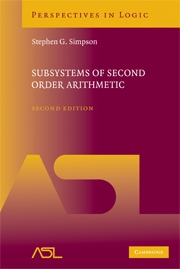# Subsystems of Second Order Arithmetic, 2nd Edition

Stephen G. SimpsonYear: 2009
ISBN-13: 9780521884396
460 pages. Hardcover.
Buy now (when ordering, include the discount code ASL2016 to receive the 25% ASL member discount)

Year: 2010
Price: \$45.00 ISBN-13: 9780521150149
464 pages. Paperback.
Buy now (when ordering, include the discount code ASL2016 to receive the 25% ASL member discount)

Foundations of mathematics is the study of the most basic concepts and logical structure of mathematics, with an eye to the unity of human knowledge. Almost all of the problems studied in this book are motivated by an overriding foundational question: What are the appropriate axioms for mathematics? Through a series of case studies, these axioms are examined to prove particular theorems in core mathematical areas such as algebra, analysis, and topology, focusing on the language of second-order arithmetic, the weakest language rich enough to express and develop the bulk of mathematics. In many cases, if a mathematical theorem is proved from appropriately weak set existence axioms, then the axioms will be logically equivalent to the theorem. Furthermore, only a few specific set existence axioms arise repeatedly in this context, which in turn correspond to classical foundational programs. This is the theme of reverse mathematics, which dominates the first half of the book. The second part focuses on models of these and other subsystems of second-order arithmetic. Additional results are presented in an appendix.

• Chapter I
• Introduction

Part A – Development of Mathematics within Subsystems of Z2: 2

• Chapter II – Recursive comprehension
• Chapter III – Arithmetical comprehension
• Chapter IV – Weak Konig’s lemma
• Chapter V – Arithmetical transfinite recursion
• Chapter VI – Pi 11 Comprehension

Part B – Models of Subsystems of Z2

• Chapter VII – Beta-models
• Chapter VIII – Omega-models
• Chapter IX – Non-omega-models
• Chapter X – Additional results